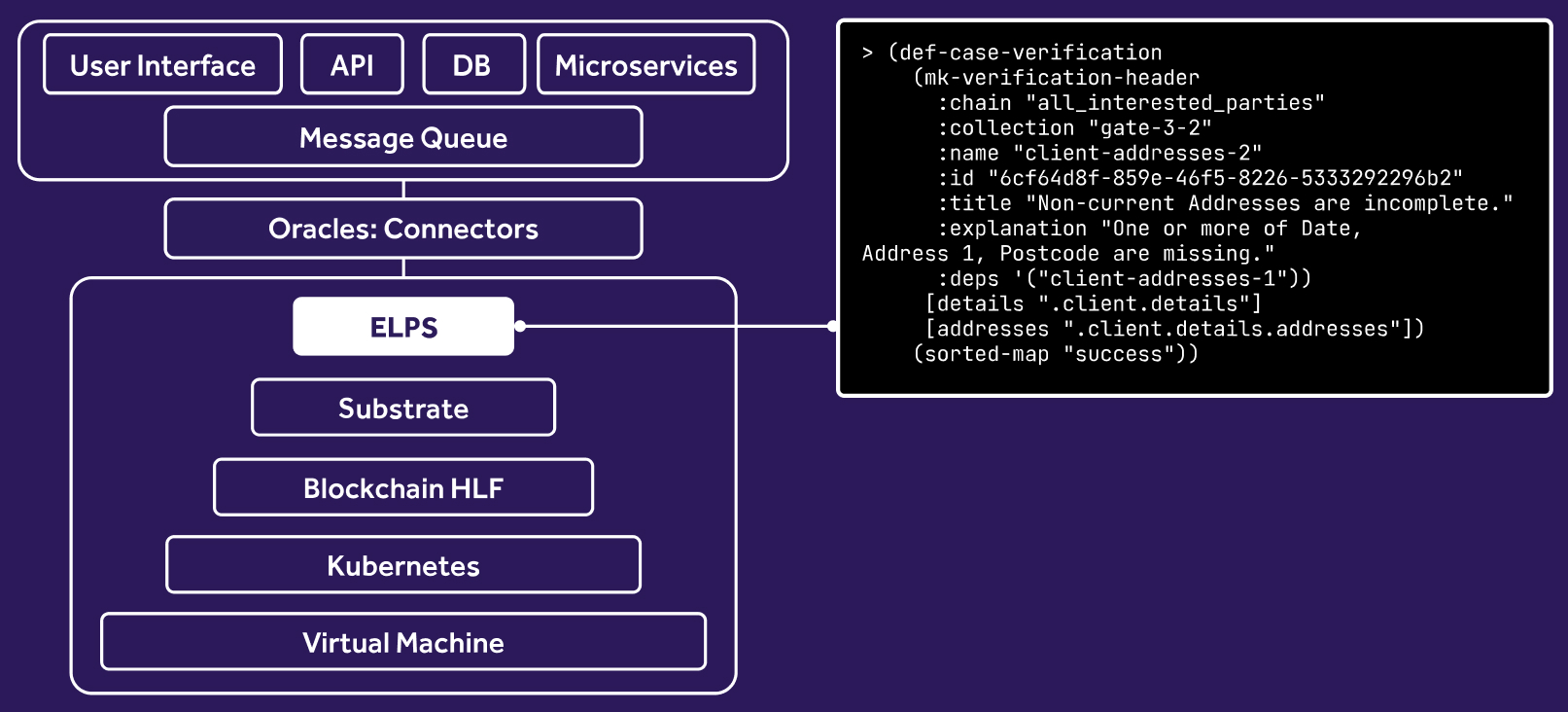# 6.3.5. Templates

Handlebars templates within ellipse.

Luther's templating library is an extension of handlebars with some additional builtins. These helper functions make writing complex templates simpler, while striving to maintain the spirit of handlebar's declarative and minimal style.

## Differences from handlebars

• Builds on the raymond Go implementation of handlebars, which aims to be feature complete with handlebarsjs v3
• New builtins: eq, len, not, and, or, gt, gte, lt, lte, times, div, mod, plus, minus, select, global
• log builtin is disabled
• printing maps is disabled (attempting to print a map will result in the string "UNPRINTABLE")

## Errors

• Where possible, the builtins will attempt to return a Go error if there was a problem parsing and rendering the template. In some cases it's not possible to distinguish whether the error was in the template itself, or the library.

## Builtins

• eq: check equality on strings and numbers.
``````template: {{eq foo 1.2}}
context: (sorted-map "foo" 1.2)
output: true
``````
• len: get the length of a sequence.
``````template: {{len array}}
context: (vector 1 2 3)
output: 3
``````
• not: Logical negation.
``````template: {{not foo }}
context: (sorted-map "foo" true)
output: false
``````
• and: Logical conjunction.
``````template: {{and test1=true test2=true test3=foo }}
context: (sorted-map "foo" false)
output: false
``````
• or: Logical disjunction.
``````template: {{and test1=foo test2=false test3=false }}
context: (sorted-map "foo" true)
output: true
``````
• gt: Check if a number is greater than another number.
``````template: {{#if (gt foo 2.2)}}yes{{/if}}
context: (sorted-map "foo" 13.1)
output: yes
``````
• gte: Check if a number is greater than or equal to another number.
``````template: {{#if (gte foo 2.2)}}yes{{/if}}
context: (sorted-map "foo" 2.2)
output: yes
``````
• lt: Check if a number is less than another number.
``````template: {{#if (lt foo 13.1)}}yes{{/if}}
context: (sorted-map "foo" 2)
output: yes
``````
• lte: Check if a number is less than or equal to another number.
``````template: {{#if (lte foo 2.2)}}yes{{/if}}
context: (sorted-map "foo" 2.2)
output: yes
``````
• times: Multiply two numbers.
``````template: {{times foo foo}}"""
context: (sorted-map "foo" 2)
output: 4
``````
• divide: Divide two numbers.
``````template: {{div foo 2}}
context: (sorted-map "foo" 5)
output: 2.5
``````
``````template: {{plus var=foo const1=2 const2=3}}
context: (sorted-map "foo" 2)
output: 7
``````
• minus: Subtract two numbers.
``````template: {{minus foo const1=2 const2=1}}
context: (sorted-map "foo" 4)
output: 1
``````
• mod: The modulo of two numbers (remainder).
``````template: {{mod foo 2}}
context: (sorted-map "foo" 3)
output: 1
``````
• select: Retrieve fields from filtered maps that are within an array of maps. It works similar to the SQL pattern of `SELECT <col> FROM <table> WHERE <cond>`, where here the table is a list of maps, the col is a field on that map whose value is retrieved, and cond is a condition that selects only the maps with a certain key-value pair.
``````template: {{#select from=metadata where="name=JWKS_URI"}}{{string_val}}{{/select}}
(vector
(sorted-map "name" "AUTH_NAME" "string_val" "Luther")
(sorted-map "name" "JWKS_URI" "string_val" "www.luthersystems.com")
(sorted-map "name" "ENABLED" "bool_val" true)))
output: www.luthersystems.com
``````
• global: create [string,string] maps in the template. This allows you to replace enum values like "INVALID_STATUS" with human readable names like "Invalid Status". It exposes an ability to create name spaces where the template can set keys on a name space using the command: `{{global "testns1" key="INVALID" val="Invalid"}}` Here the namespace is "testns1", and the command is inserting a value "Invalid" for key "INVALID" into that namespace. To retrieve the previously inserted value, use the same command without the put: `{{global "testns1" key=tKey}}` In this example, `tKey` is a variable available within the context.
``````template: {{global "testns1" key="INVALID" val="Invalid"}}{{global"testns1" key=tKey}}
context: (sorted-map "tKey" "INVALID")
output: Invalid
``````
• is-after: Check if a given date is after a reference date.
``````template: {{is-after "2020-01-01" "2019-10-01"}}
output: true
``````
• date-diff-month: Calculate the difference between two dates in months. Always rounded up.
``````template: {{date-diff-month "2020-01-01" "2019-01-02"}}
output: 12
``````
• date-add-months: Add X months to a given date. Supports negative months.
``````template: {{date-add-months "2020-01-01" -1}}
output: 2019-12-01
``````